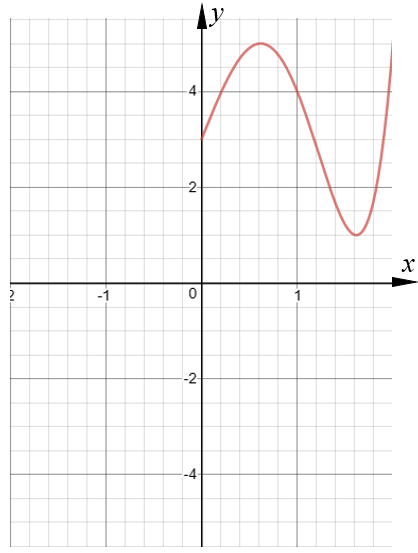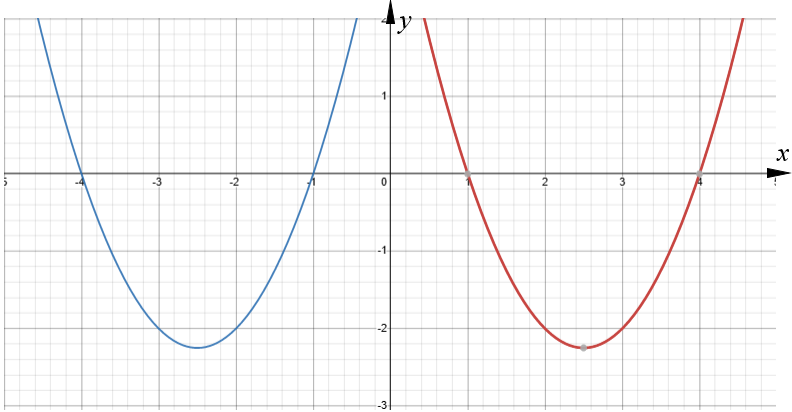# Exam-Style Questions.

## Problems adapted from questions set for previous Mathematics exams.

### 1.

GCSE Higher

The graph of the curve A with equation $$y=f(x)$$ is transformed to give the graph of the curve B with equation $$y=5-f(x)$$.

The point on A with coordinates (3, 9) is mapped to the point W on B.

Find the coordinates of W.

### 2.

GCSE Higher

The graph of the curve with equation y = $$f(x)$$ is shown on the grid below.(a) On the grid above, sketch the graph of the curve with equation $$y = f(–x)$$

The red curve with equation $$y = x^2-5x+4$$ is transformed by a translation to give the blue curve such that the point (2.5, -2.25) on the red curve is mapped to the point (-2.5, -2.25) on the blue curve.(b) Find an equation for the blue curve.

### 3.

GCSE Higher

The graph of the following equation is drawn and then reflected in the x-axis

$$y = 2x^2 - 3x + 2$$

(a) What is the equation of the reflected curve?

The original curve is reflected in the y-axis.

(b) What is the equation of this second reflected curve?

### 4.

IB Standard

$$f$$ and $$g$$ are two functions such that $$g(x)=3f(x+2)+7$$.

The graph of $$f$$ is mapped to the graph of $$g$$ under the following transformations:

A vertical stretch by a factor of $$a$$ , followed by a translation $$\begin{pmatrix}b \\c \\ \end{pmatrix}$$

Find the values of

(a) $$a$$;

(b) $$b$$;

(c) $$c$$.

(d) Consider two other functions $$h$$ and $$j$$. Let $$h(x)=-j(2x)$$. The point A(8, 7) on the graph of $$j$$ is mapped to the point B on the graph of $$h$$. Find the coordinates of B.

If you would like space on the right of the question to write out the solution try this Thinning Feature. It will collapse the text into the left half of your screen but large diagrams will remain unchanged.

The exam-style questions appearing on this site are based on those set in previous examinations (or sample assessment papers for future examinations) by the major examination boards. The wording, diagrams and figures used in these questions have been changed from the originals so that students can have fresh, relevant problem solving practice even if they have previously worked through the related exam paper.

The solutions to the questions on this website are only available to those who have a Transum Subscription.

Exam-Style Questions Main Page

Search for exam-style questions containing a particular word or phrase:

To search the entire Transum website use the search box in the grey area below.